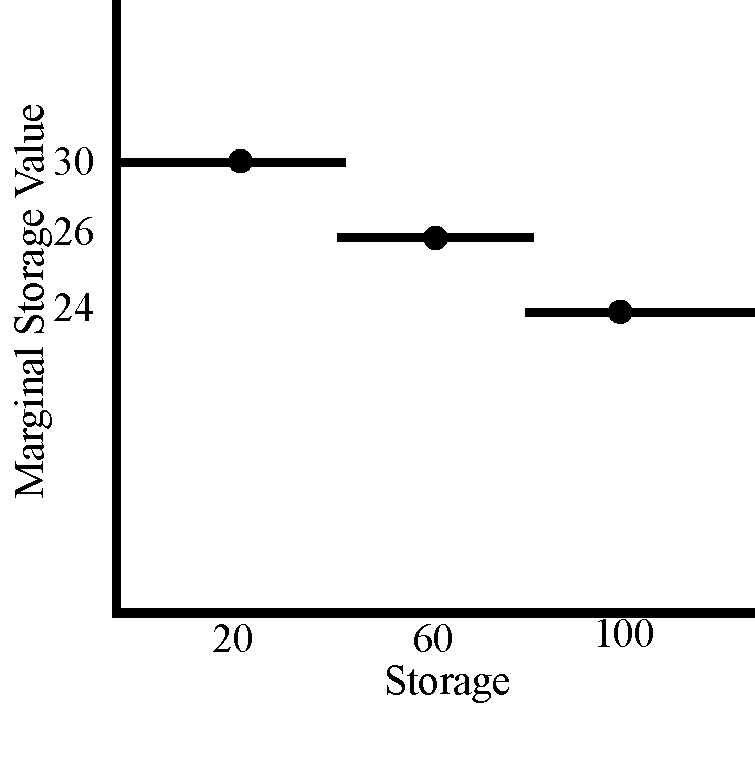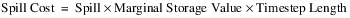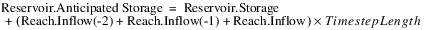Future Value
The methods in this category are used to determine the future value of the energy that would have been generated by the water that was lost through the spillway.None
None is the default method for the Future Value category. No calculations are performed by this method.
There are no slots specific to this method.Cumulative Storage Value Table
Slots Specific to This Method
Marginal Storage Value Table
Type: Table
Units: Volume vs. \$per Energy
Description: Storage versus marginal value per unit energy
Information: This table should be increasing in storage, and usually decreasing in marginal value
I/O: Required input
Spill Cost
Type: Series Slot
Units: \$
Description: Future cost of energy lost due to spilled water
Information:
I/O: Output only
Anticipated Storage
Type: Series
Units: Volume
Description: The combination of Storage in the reservoir at the given timestep plus any flow (converted volume) that is in transit to the reservoir
Information: This slot represents the storage including any lagged flows that are already in a linked upstream reach, which will reach the reservoir at a later timestep. If there are no lagged reaches between this reservoir and the next upstream reservoir, Anticipated Storage will equal Storage. It is this storage value that will be used to calculate Cumulative Storage Value.
I/O: Output only
Cumulative Storage Value
Type: Series Slot
Units: \$
Description: Represents the future energy value of the current Anticipated Storage
Information:
I/O: Output only
Cumulative Storage Value Table
Type: Table
Units: Volume vs. \$
Description: Anticipated Storage and cumulative value used to calculate the Cumulative Storage Value as a function of Anticipated Storage
Information: This table should be increasing in storage and usually increasing in cumulative storage value.
I/O: Required input either by the user or automatically generated by RiverWare if the Cumulative Storage Value Table Automation method is selected.
Method Details
This method uses the Marginal Storage Value Table and the calculated Spill and Turbine Release to compute the Spill Cost. These are the only calculations performed by this method.
The correct marginal value is found from the current storage in the reservoir. If the current storage is than the midpoint between the first and second storage table values, the first marginal value is used. The second marginal value is used for a current storage above that midpoint to the midpoint between the second and third storage table values. The last marginal value is used for any current storage above the midpoint between the second-to-last and the last storage table value. Table 25.3 is an example.

Table 25.3  Marginal Value Table
Storage
Marginal Value
20
30
60
26
100
24Assume that the current storage is 39. Therefore, this method would use 30 as the marginal value for use in the next computation. Assume that the current storage is 41. Therefore, this method would use 26 as the marginal value for use in the next computation.
Use of a table in this fashion is unique to this method.
Spill Cost is computed by the following equation:The Cumulative Storage Value computation begins by first calculating Anticipated Storage. This is the sum of the reservoir Storage plus any flow already in transit to the reservoir in an upstream lagged reach. For example, assume a reservoir’s Inflow slot is linked to a reach with a three-hour lag time. In an run with a 1 Hour timestep, the reservoir’s Anticipated Storage would be calculated as follows:If there are no lagged reaches between the reservoir and the next upstream reservoir, then Anticipated Storage will simply equal Storage.
The Cumulative Storage Value is then computed by interpolating from the Cumulative Storage Value Table using the calculated Anticipated Storage value.
Revised: 08/02/2021